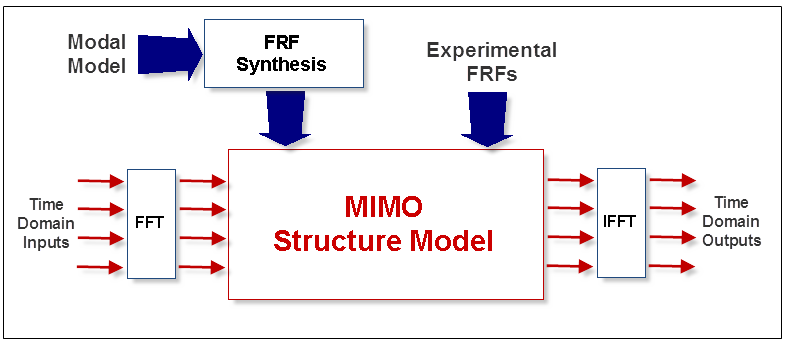# Calculating Outputs From Inputs

Outputs can be calculated from Inputs & Transfer Functions in three different ways;

1. Output Time Waveforms or Fourier spectra from Input Time Waveforms or Fourier spectra & Transfer Functions

2. Cross spectra between Inputs & Outputs.

3. Output Auto spectra or PSDs from Auto spectra or PSDs & Transfer Functions

## Output time Waveforms or Fourier Spectra

Output Fourier spectra are calculated from Transfer Functions and Input Fourier spectra using the formula;

{X(w)} = [H(w)] {F(w)}

where:

{F(w)} - Fourier spectra of Inputs (m - vector)

[H(w)] - Transfer Function matrix (n by m)

{X(w)} - Fourier spectra of Outputs (n - vector)

m - number of Inputs

n - number of Outputs

w - frequency variable

• If Input Time Waveforms are provided as a Source, they are transformed to Fourier spectra before using the above equation

• The calculated Output Fourier spectra are then transformed to Output Time Waveforms

The following block diagram depicts the Output calculation when the Inputs are provided as time waveforms, and either a Modal Model or Experimental FRFs are also provided for the Transfer Function matrix.MIMO Output Calculation.

## Cross Spectra

Cross spectra between Inputs & Outputs are calculated from Input Auto spectra & Transfer Functions using the formula;

[{X(w)}{F(w)}t] =  [H(w)] [{F(w)}{F(w)}t]

where:

[{F(w)}{F(w)}t] - Input Auto spectrum matrix (m by m)

[H(w)] - Transfer Function matrix (n by m)

[{X(w)}{F(w)}t] - Cross spectrum matrix between Inputs & Outputs (n by m)

m - number of Inputs

n - number of Outputs

w - frequency variable (radians per second)

t - denotes transposed conjugate

## Output Auto Spectra

Output Auto spectra or PSDs are calculated from Input Auto spectra or PSDs & Transfer Functions using the formula;

[{X(w)}{X(w)}t] = [H(w)] [{F(w)}{F(w)}t] [H(w)]t

where:

[{F(w)}{F(w)}t] - Input Auto spectrum matrix (m by m)

[H(w)] - Transfer Function matrix (n by m)

[{X(w)}{X(w)}t] - Output Auto spectrum matrix (n by n)

m - number of Inputs

n - number of Outputs

w - frequency variable (radians per second)

t - denotes transposed conjugate

Only the diagonal elements of the Output Auto spectrum matrix are calculated.